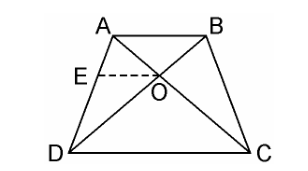Guru

# ABCD is a trapezium in which AB || DC and its diagonals intersect each other at the point O. Show that AO/BO = CO/DO. Q.9

• 0

The chapter from triangles how i solve the problem from exercise 6.2 of math subject, how i solve this problem in easy way ABCD is a trapezium in which AB || DC and its diagonals intersect each other at the point O. Show that AO/BO = CO/DO.

Share

1. Given, ABCD is a trapezium where AB || DC and diagonals AC and BD intersect each other at O.We have to prove, AO/BO = CO/DO

From the point O, draw a line EO touching AD at E, in such a way that,

EO || DC || AB

In ΔADC, we have OE || DC

Therefore, By using Basic Proportionality Theorem

AE/ED = AO/CO ……………..(i)

Now, In ΔABD, OE || AB

Therefore, By using Basic Proportionality Theorem

DE/EA = DO/BO…………….(ii)

From equation (i) and (ii), we get,

AO/CO = BO/DO

⇒AO/BO = CO/DO

Hence, proved.

• 0